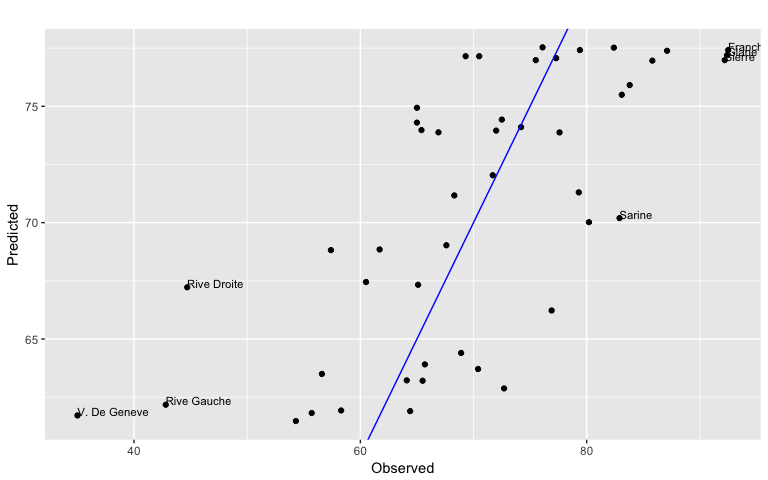# Exploratory Data Analysis Using Random Forests

#### 2017-03-05

This package allows you to compute the partial dependence of covariates, compute permutation importance, and case/observation-level distance between data points given a fitted random forest from the following packages (outcome variable types supported in parenthesis): party (multivariate, regression, and classification), randomForestSRC (regression, classification), randomForest (regression and classification), and ranger (classification and regression).

The function partial_dependence outputs a data.frame of class pd. By default all of the supported random forest classifiers will return probabilities.

The vars argument is a character vector which gives the features for which the partial dependence is desired.

library(edarf)

data(iris)
library(party)
## Loading required package: grid
## Loading required package: mvtnorm
## Loading required package: modeltools
## Loading required package: stats4
## Loading required package: strucchange
## Loading required package: zoo
##
## Attaching package: 'zoo'
## The following objects are masked from 'package:base':
##
##     as.Date, as.Date.numeric
## Loading required package: sandwich
fit <- cforest(Species ~ ., iris, controls = cforest_unbiased(mtry = 2))
pd <- partial_dependence(fit, vars = "Petal.Width", n = c(10, 25))
print(pd)
##    Petal.Width    setosa versicolor  virginica
## 1    0.1000000 0.6971284  0.2349063 0.06796526
## 2    0.3666667 0.6914523  0.2398904 0.06865734
## 3    0.6333333 0.3007795  0.5681631 0.13105747
## 4    0.9000000 0.3007795  0.5681631 0.13105747
## 5    1.1666667 0.2990429  0.5697648 0.13119223
## 6    1.4333333 0.3100852  0.5263477 0.16356711
## 7    1.7000000 0.3174794  0.1946512 0.48786943
## 8    1.9666667 0.3127924  0.1729954 0.51421220
## 9    2.2333333 0.3126790  0.1728630 0.51445792
## 10   2.5000000 0.3126790  0.1728630 0.51445792

The parameter n is a numeric vector of length two, which controls via its first element the size of the grid to evaluate the columns specified by the vars argument at, and the second element gives the number of rows of the other variables to sample when marginalizing the prediction function. All the actual computation is performed in the mmpf package using the function marginalPrediction. Additional arguments can be passed to this function by name (i.e., using ...).

Output from partial_dependence can be visualized using plot_pd, which uses ggplot2 to create simple visualizations. In this simple case a line plot for each class.

plot_pd(pd)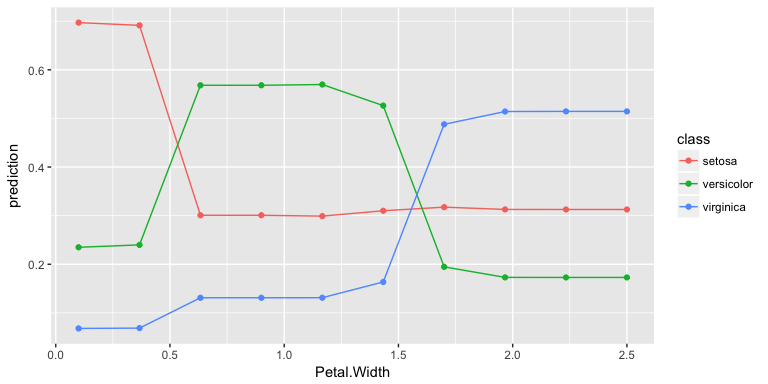The partial_dependence method can also either return interactions (the partial dependence on unique combinations of a subset of the covariates) or a list of bivariate partial dependence estimates (when multiple covariates are specified in vars but interaction = FALSE).

pd_list <- partial_dependence(fit, c("Petal.Width", "Petal.Length"), n = c(10, 25), interaction = FALSE)
plot_pd(pd_list)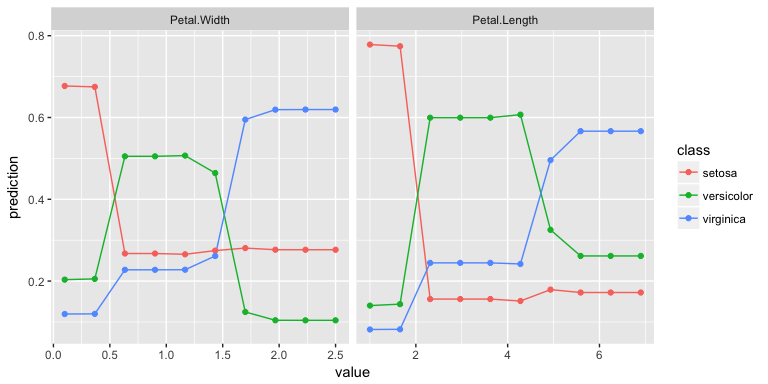pd_int <- partial_dependence(fit, c("Petal.Length", "Petal.Width"), n = c(10, 25), interaction = TRUE)
plot_pd(pd_int)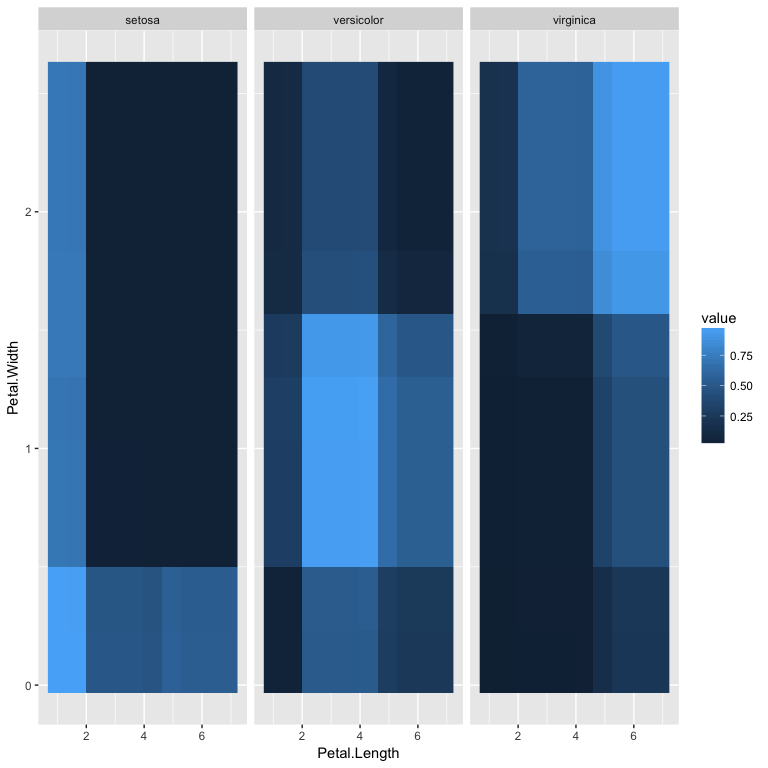When an interaction is computed the facet argument is used to construct plots wheren the feature not set to be the facet is shown conditional on a particular value of the facet feature. This works best when said feature is an integer or a factor.

variable_importance can be used to compute the permutation importance of covariates. A particular covariate, or covariates, as specified by the vars parameter, are randomly shuffled, and predictions are computed. The aggregate permutation importance is the mean difference between the unpermuted predictions and the predictions made when the covariates specified in vars are permuted. If unspecified vars is set to all of the covariates used in the fit.

imp <- variable_importance(fit, nperm = 10)
plot_imp(imp)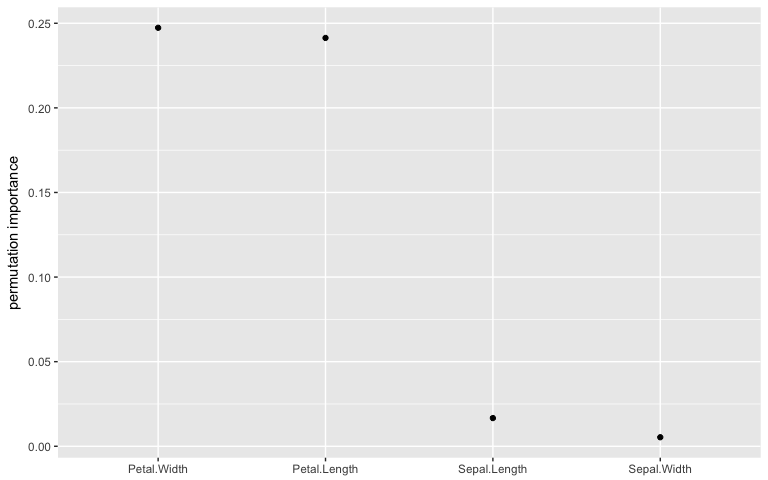The joint permutation importance of multiple covariates can be computed by setting interaction = TRUE. variable_importance relies on permutationImportance in mmpf for computation, and additional arguments which allow the computation of local or class-specific importance, as well as the importance under arbitrary loss and contrast functions, can be passed by name to permutationImportance.

extract_proximity extracts or computes a matrix which gives the co-occurence of data points in the terminal nodes of the trees in the fitted random forest. This can be used to visualize the distance between data points in the model.

This matrix is too large to be visualized. plot_prox takes a principal components decomposition of this matrix using prcomp, and plots it using a biplot. Additional arguments allow other covariates to be mapped onto the size of the points, their color (shown), or their shape.

fit <- cforest(Species ~ ., iris, control = cforest_unbiased(mtry = 2))
prox <- extract_proximity(fit)
pca <- prcomp(prox, scale = TRUE)
plot_prox(pca, color = iris$Species, color_label = "Species", size = 2)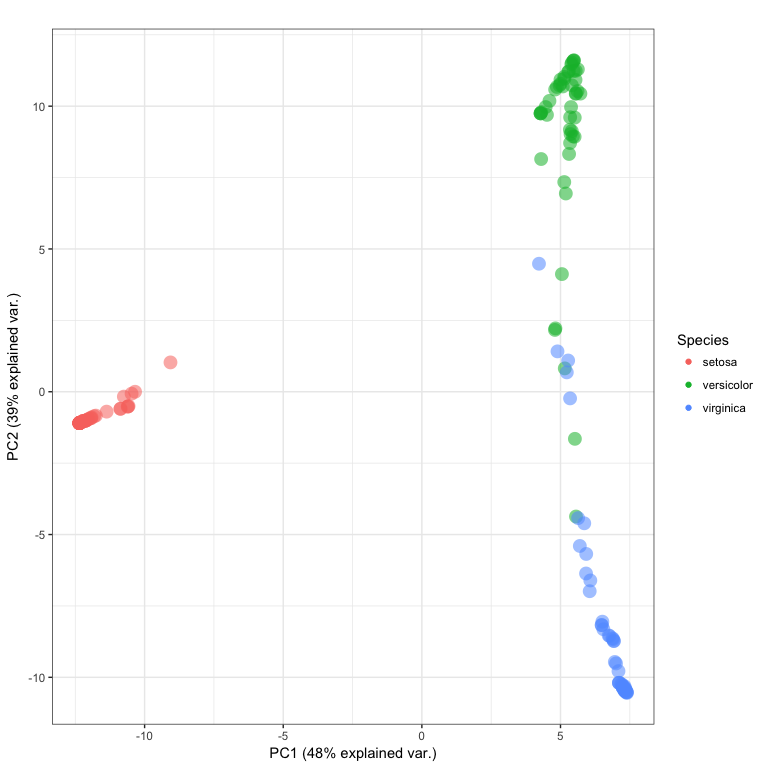The function plot_pred makes plots of predictions versus observations and has the additional feature of being able to “tag” outliers, and assign them labels. fit <- cforest(Fertility ~ ., swiss) pred <- as.numeric(predict(fit, newdata = swiss)) plot_pred(pred, swiss$Fertility,
outlier_idx = which((pred - swiss$Fertility)^2 > var(swiss$Fertility)),
labs = row.names(swiss))